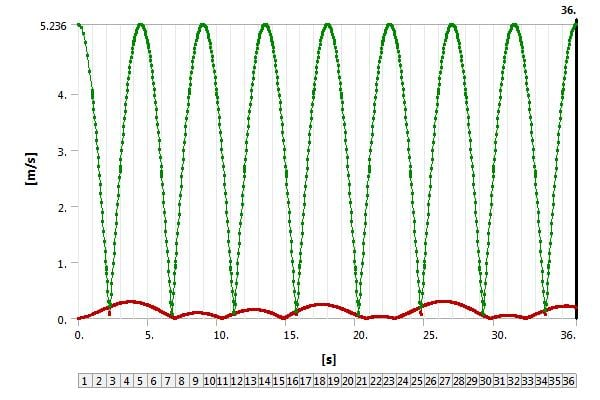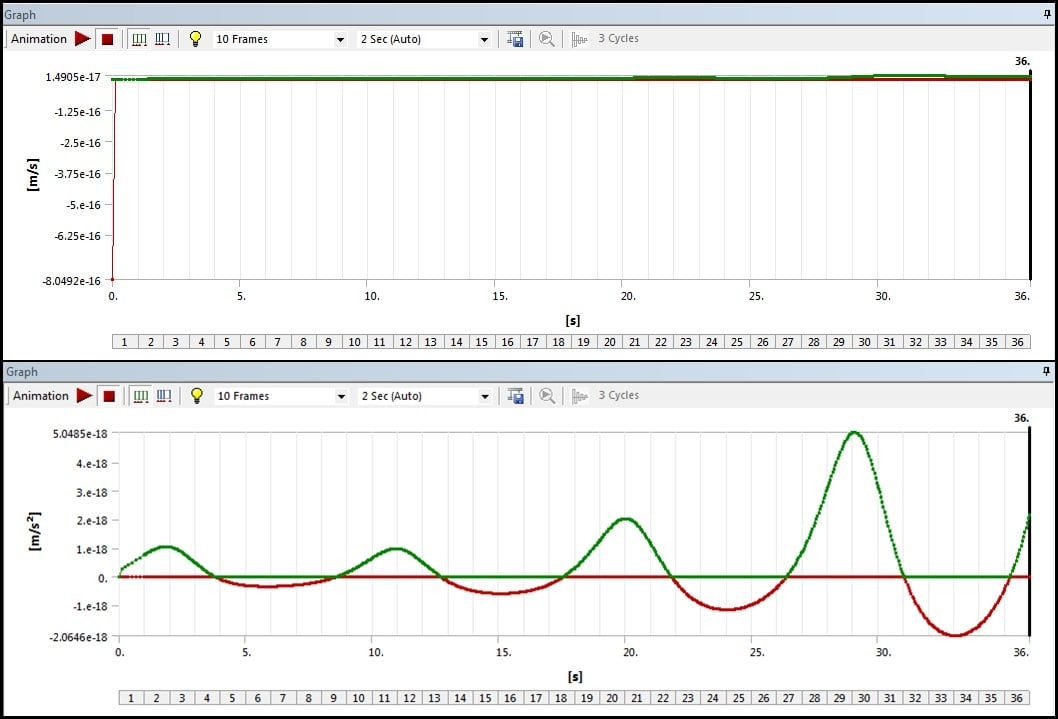## General Mechanical

•Naveed
Subscriber

I have 2 DOF spring mass system. I applied remote displacement to the lower mass. My input is sinusoidal wave. But the Total Deformation, Total Velocity and Total Acceleration graphs are showing only positive values. Why? Outputs should also be sinusoidal. Isn't it? Please guide. Thanks in advance.•Rob
Ansys Employee

Check what you're monitoring as velocity magnitude will always be positive. How does the frequency compare to the input signal?

As an aside, adding a tag to show which code you used will help the community assist you.

•Naveed
Subscriber

Rwoolhou, thanks for your answer. I am new to ANSYS. In mechanical, from the solutions (in the outline tab) I added "total velocity". Frequency is constant. My input remote displacement is: 7.5*sin(40*time).

I made a geometry. No code is used here.

•Rob
Ansys Employee

Thanks, by code I mean which of Mechanical, Fluent, CFX, HFSS etc did you use: sounds like it's Mech so I'll tag this for the community.

I suspect total velocity will always be a positive, but the vector will change.

•peteroznewman
Subscriber

Plot Directional Velocity instead of Total Velocity to get a sinusoidal output for a sinusoidal input displacement.

•Naveed
Subscriber

Thanks Peter. I tried that and it is giving me some weird output. Any idea what's happening?
Although directional acceleration looks acceptable.•peteroznewman
Subscriber

The top graph is plotting a value of zero with some numerical error mixed in.
What is the output scoped to and what are the boundary conditions?

•Naveed
Subscriber

Thanks a lot Peter. After selecting directional velocity I need to specify the axis. After selecting Y axis it is showing the results as were expected. Thanks man.